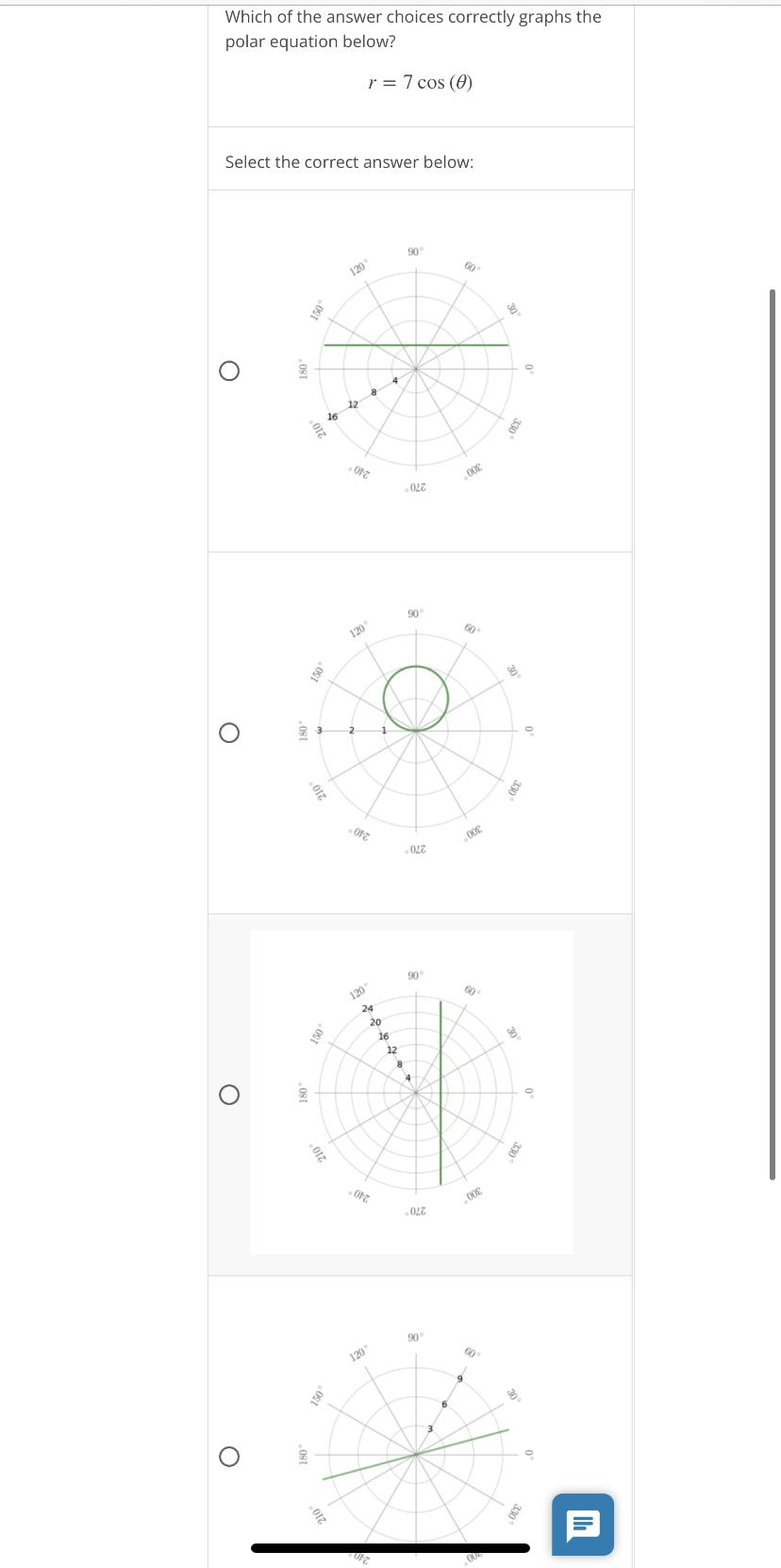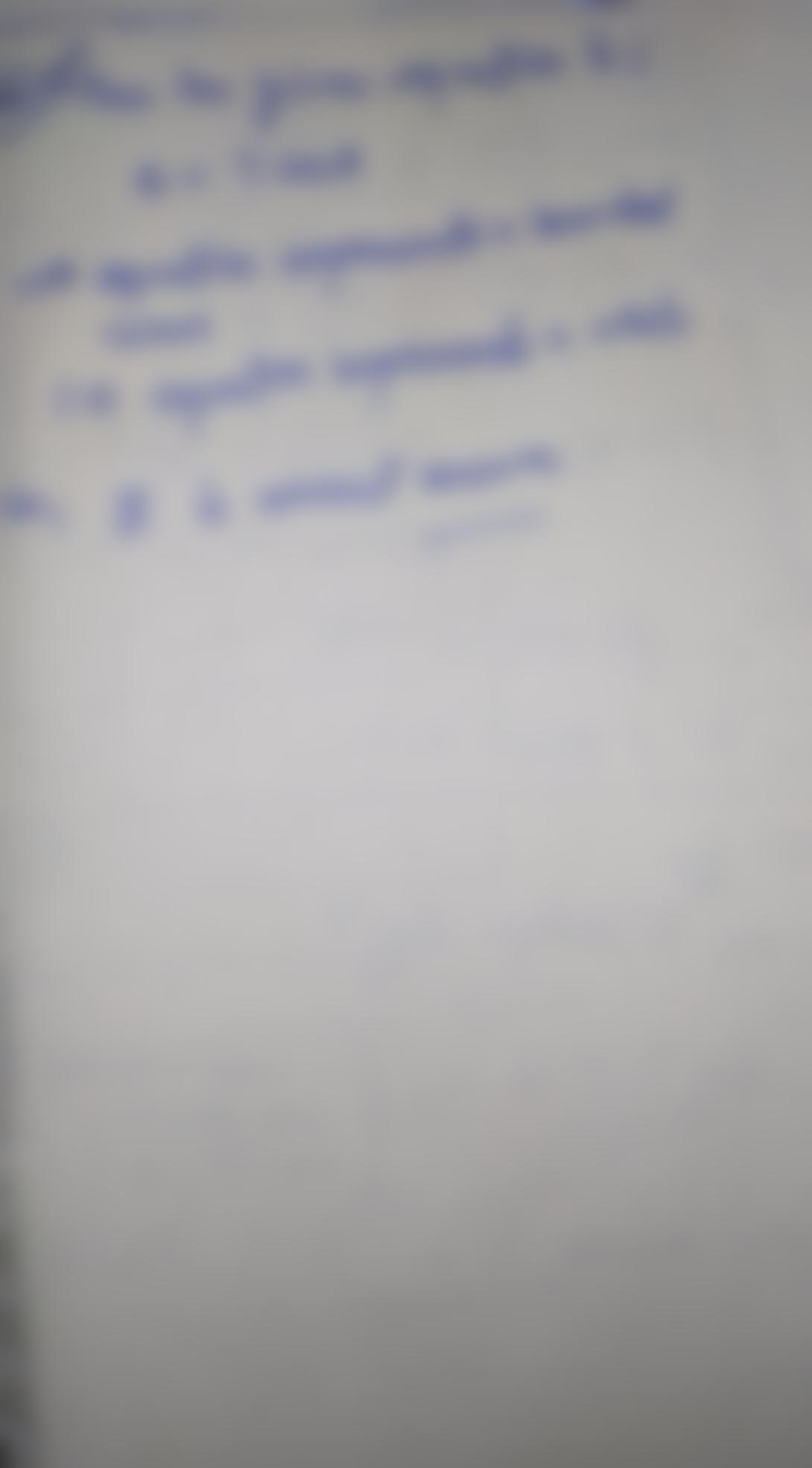Question:

Which of the answer choices correctly graphs the polar equation below? r = 7 cos (0) Select the correct answer below: 90 50 120Which of the answer choices correctly graphs the polar equation below? r = 7 cos (0) Select the correct answer below: 90 50 120 O 4 12 16 210 09 012 ODE .022 90 120 o 2 1 210 OPZ 005 .022 30 120 24 20 16 12 8 4 O 210 055 012 00€ 022 30 120 6 3 210 3